Paclet Resource

Wolfram

# QuantumFramework

Perform analytic and numeric computation in Quantum Information Theory

Contributed By: Wolfram Research, Quantum Computation Framework team

Quantum Computing is an emerging technology that could revolutionize how computations are performed in the future. It operates based on the law of quantum mechanics - a radically different law of physics from the one our current computers are based on. A quantum computer may be able to solve problems that are difficult to solve using current computers such as breaking encryption, simulating a quantum system, or enhancing machine learning algorithms.

The Wolfram Language Quantum Computation Framework performs high-level analytic and numeric computations in Quantum Information Theory, allowing simulation of quantum circuits and quantum algorithms. Starting from discrete quantum mechanics, this framework can work with quantum states and quantum operators, implements measurements, performs basis manipulation, computes measure entanglement, and more. The semantics is very intuitive and it is equipped with various named states and operators such as Bell states, Pauli operators, universal quantum gates, and others. Thus, making it easy for people to simulate a quantum computer using the Wolfram Language.## Details

This paclet works with V12.3 and more of Woflram Language Mathematica.

## Examples

### Quantum Basis and State (19)

In our Quantum Framework, a quantum state is defined with respect to a QuantumBasis. For pure states, the given input can be a list of amplitudes, or an association with keys corresponds to basis elements and values as amplitudes.

Define a 2D quantum basis (computational):

 In:=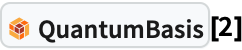Out=Given the basis dimension n, the basis elements will be indexed by ket with i=0,1,...,n-1

 In:=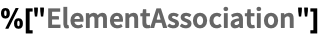Out=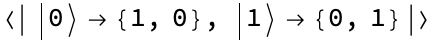Use a sequence as QuantumBasis[n,m], for n qudits of m-dimensional (overall dimension will be nm). For example, let us define a 2×2×2 dimensional quantum basis (three qubits):

 In:=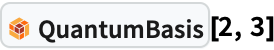Out=In:=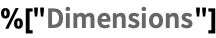Out=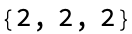Use a list as QuantumBasis[{n1,n2,...,nm}] for n1×n2×n2×...×nm dimensional Hilbert space of m qudits. For example, define a 3×5 dimensional quantum basis (two qudits):

 In:=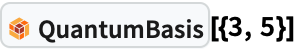Out=In:=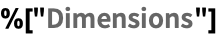Out=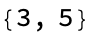A basis can also be defined by an association with the basis element names as keys, and corresponding vectors as values.

 In:=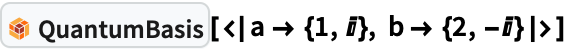Out=There are many named-basis built in the quantum framework, {"Computational", "PauliX", "PauliY", "PauliZ","Fourier", "Identity","Schwinger", "Pauli", "Dirac", "Wigner"}:

 In:=Out=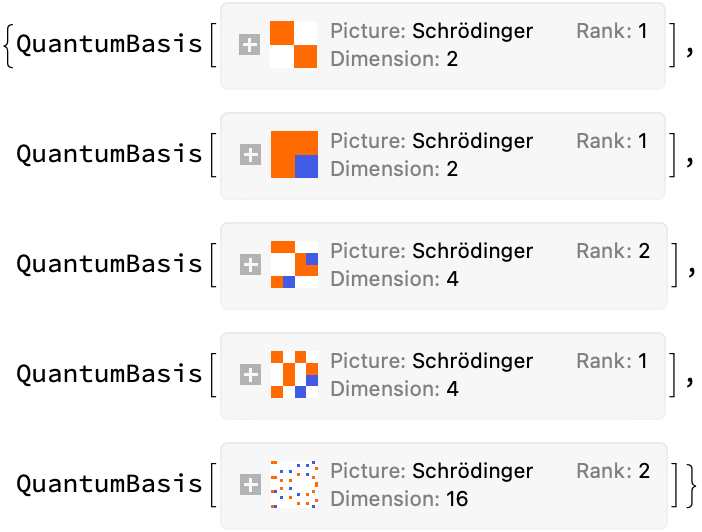After a basis object has been defined, it is straightforward to use it to construct quantum states and operators. A quantum state is represented by QuantumState object and a quantum operator is represented by QuantumOperator.

For pure quantum states, a vector with elements as amplitudes, and the corresponding basis should be given in this format: QuantumState[amp_List,QuantumBasis[args]]. With no basis specified, the default basis will be the computational basis whose dimension depends on the amplitude vector.

Define a pure 2-dimensional quantum state (qubit) in the Pauli-X basis:

 In:=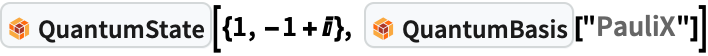Out=In:=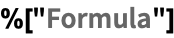Out=If the basis is not specified, the default is the computational basis of 2n dimension (n qubits):

 In:=Out=Out=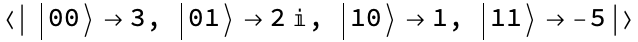If the vector has more than 2 elements, it is interpreted as a n-qubit state (by right-padding of zeros), unless the dimension is specified.

 In:=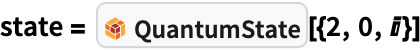Out=In:=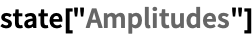Out=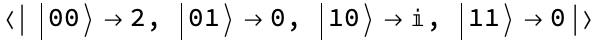Same amplitude vector, but this time the dimension is specified:

 In:=Out=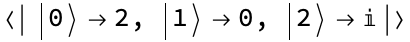Many named states are available for easy access:

 In:=Out=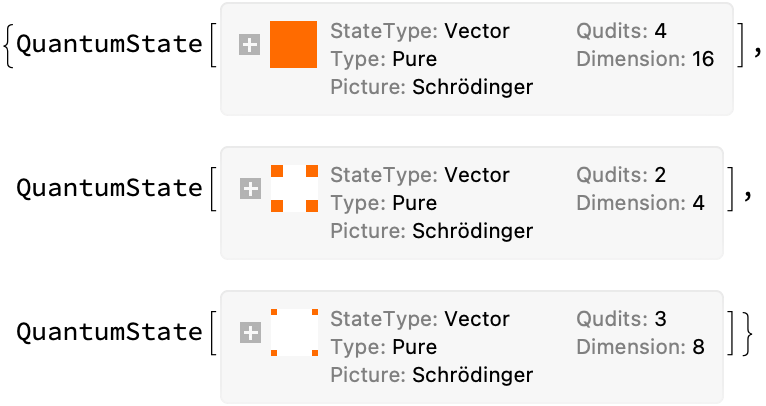Using associations, one can create a superposition of states, with keys as a list of corresponding indexes, and values as amplitudes. For example, let us create a superposition of 3 qubits (i.e., QuantumBasis[2,3]) as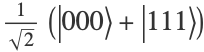:

 In:=Out=In:=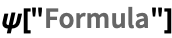Out=In:=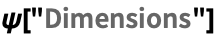Out=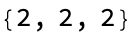A different way of creating superposition is simple adding two quantum state objects. For example, the previous state can be constructed as follows, too:

 In:=Out=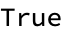Once a built-in basis is specified, amplitudes correspond to the basis elements. For example, let's use Bell basis:

 In:=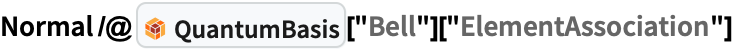Out=In:=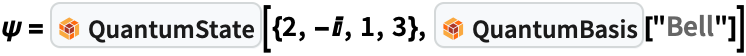Out=In:=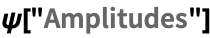Out=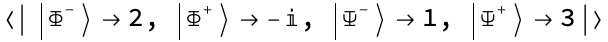We can also define a state by inputting a density matrix:

 In:=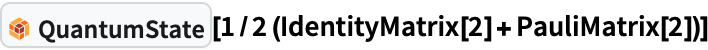Out=For pure states, one can get the corresponding normalized state vector:

 In:=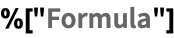Out=Define a generic Bloch vector:

 In:=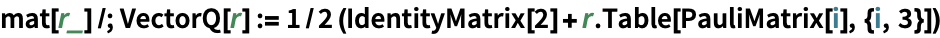In:=Out=Test if it is a mixed state:

 In:=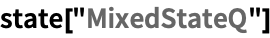Out=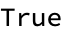Calculate Von Neumann Entropy:

 In:=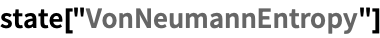Out=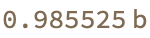Purity:

 In:=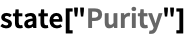Out=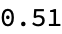A pure state (by normalizing the vector r):

 In:=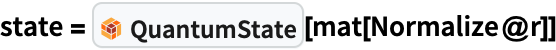Out=Purity:

 In:=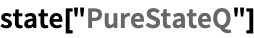Out=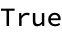Calculate Bloch Spherical Coordinates

 In:=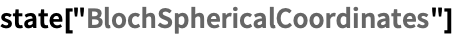Out=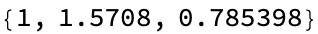A matrix that is not positive semi-definite (cannot be a density matrix in standard QM, but in ZX-formalism we can have situations like this):

 In:=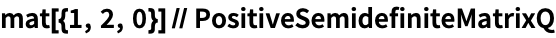Out=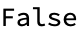A non positive-semidefinite matrix as state:

 In:=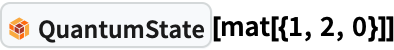Out=